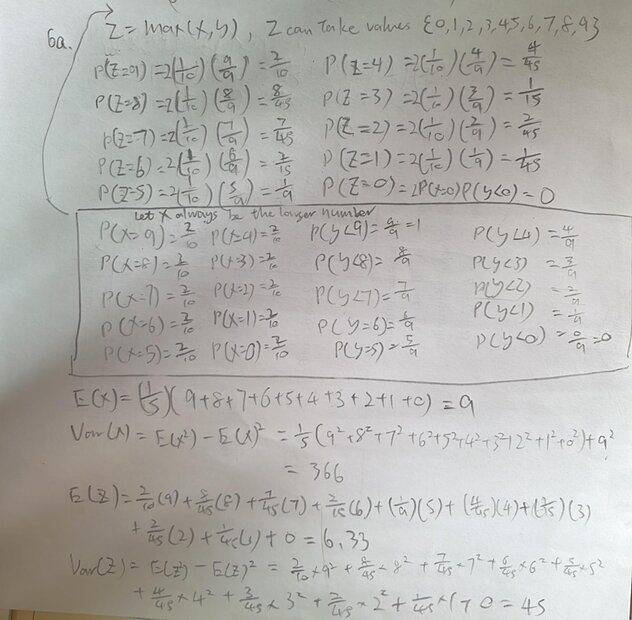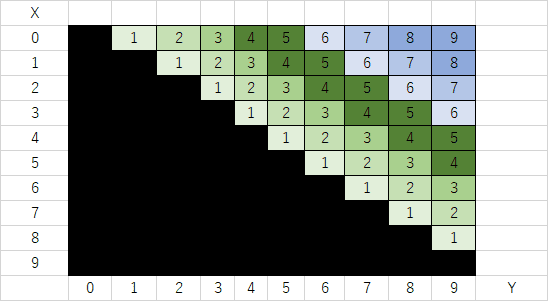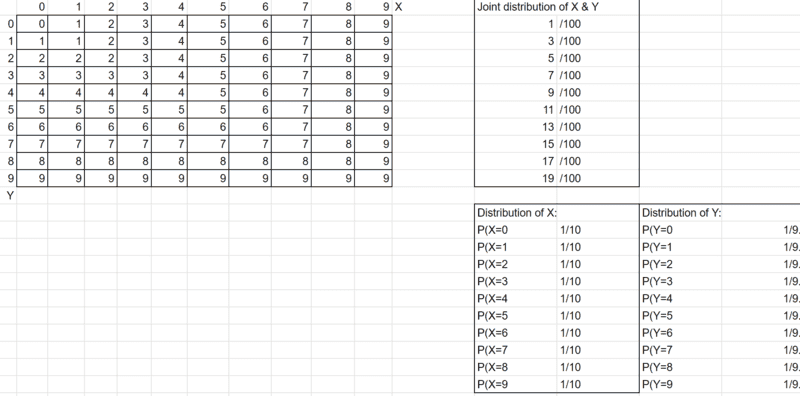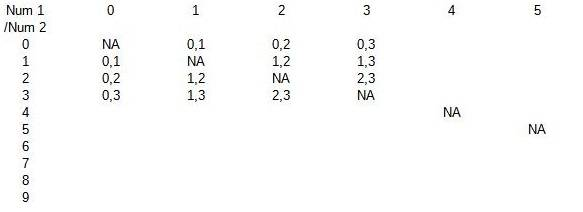# Finding the distribution of random variables

• LokLe
Your distribution of Y adds up to more than 1, so we can see it must be wrong. Furthermore, it does not make sense that the probabilities of the X values are equal. Can the maximum being 1 (only two ways to get that) really be as likely as the maximum being 9 (more than two ways to get that)?Your distribution of Y adds up to more than 1, so we can see it must be wrong. Furthermore, it does not make sense that the probabilities of the X values are equal. Can the maximum being 1 (only two ways to get that) really be as likely as the maximum being 9 (more than two ways to get that)?f

#### LokLe

Homework Statement
In a box of ten tickets numbered 0, 1, 2,...,9. You pick at random 2 tickets from this box. Let
X is the larger number in these two tickets, and Y be the smaller one.
(a) Find the distributions of X, Y and their joint distribution.
(b) Find the distribution of Z=X-Y.
(c) Compute E(X), Var(X), E(Z) and Var(Z).
Relevant Equations
None
Hi. I have found the answer to a and c (I don't know whether it is correct) but I do not know what I should find in question b.Is my method correct and how should I solve part b?

I made a 10x10 table and counted the occurrence of each max and min. There is a simple pattern.
I don't get the same answers in (a). Can you explain what your logic is? I have trouble reading your work, but it looks like your probabilities add up to more than 1. [EDIT: It looks like I agree with the numbers at the top but not with the numbers in the box.]

Last edited:
•anuttarasammyak
I also made a table with number Z in the cells.Last edited:
•WWGD and FactChecker
I made a 10x10 table and counted the occurrence of each max and min. There is a simple pattern.I tried to correct the mistakes by making a 10x10 table.

We have the same chance of getting a number from 0 to 9, so the distribution of (X=x) should be 1/10.
Since we have already picked a number for X, (Y=y) should be 1/(10-1) = 1/9?

I also made a table with number Z in the cells.View attachment 298596
I understand part b now. Thank you!

I tried to correct the mistakes by making a 10x10 table.

We have the same chance of getting a number from 0 to 9, so the distribution of (X=x) should be 1/10.
Since we have already picked a number for X, (Y=y) should be 1/(10-1) = 1/9?
You pick up two tickets AT A TIME. Then you read them to call larger one Y and smaller one X.
All the possible cells in the table have an equal probability.

You pick up two tickets AT A TIME. Then you read them to call larger one Y and smaller one X.
All the possible cells in the table have an equal probability.
Oh so X=x and Y=y have an equal distribution. I will correct it. Thank you.

Oh so X=x and Y=y have an equal distribution. I will correct it. Thank you.
I would say all the cells (x,y) x<y are equal in probability or in distribution.

•LokLe
Then you read them to call larger one Y and smaller one X.
You got that backwards.

•anuttarasammyak

View attachment 298597

I tried to correct the mistakes by making a 10x10 table.

We have the same chance of getting a number from 0 to 9, so the distribution of (X=x) should be 1/10.
Since we have already picked a number for X, (Y=y) should be 1/(10-1) = 1/9?
Your distribution of Y adds up to more than 1, so we can see it must be wrong. Furthermore, it does not make sense that the probabilities of the X values are equal. Can the maximum being 1 (only two ways to get that) really be as likely as the maximum being 9 (more than two ways to get that)?
I would start with a 10x10 table of the two numbers drawn. In each cell, I would put the Y,X values and see the pattern. Each combination of two numbers has equal probability, so we can just count how many of the cells are in particular events like X=3 or Y=5. I think you had the right pattern in the top section of your original post, but it is hard to read and unexplained.Last edited: